# ▸ Microsoft Excel | LinkedIn Skill Assessment Quiz Solutions

Recommended Microsoft Excel Courses:

1. Some of your data in Column C is displaying as hashtags (#) because the column is too narrow. How can you widen Column C just enough to show all the data?

• Right-click column C, select Format Cells, and then select Best-Fit.
• Right-click column C and select Best-Fit.
• Double-click column C.
• Double-click the vertical boundary between columns C and D.

1. Which two functions check for the presence of numerical or nonnumerical characters in cells?

• ISNUMBER and ISTEXT
• ISNUMBER and ISALPHA
• ISVALUE AND ISNUMBER
• ISVALUE and ISTEXT

1. If you drag the fill handle (lower-right corner) of cell A2 downward into cells A3, A4, and A5, what contents will appear in those cells?• Jan, Jan, Jan
• Feb, Mar, blank cell
• Feb, Mar, Apr
• FEB, MAB, APR

1. If cell A3 contains the text THE DEATH OF CHIVALRY, what will the function =PROPER(A3) return?

• the death of chivalry
• The death of Chivalry
• THE DEATH OF CHIVALRY
• The Death Of Chivalry

1. In the worksheet below, you want to use Data > Subtotal to show a subtotal value per sport. What must you do BEFORE applying the Subtotal function?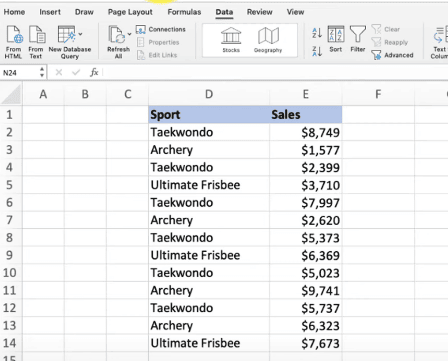• Sort by the data in Column E.
• Format the data in Column D.
• Sort by the data in Column D.
• Format the data in Column E.

1. You want to apply subtotals per sport in the data below. What should you do before applying subtotals?• Format the data in column E.
• Format the data in column D.
• Sort the data in column D.
• Sort the data in column E.

1. When editing a cell, what do you press to cycle between relative, mixed, and absolute cell references?

• Alt+F4 (Windows) or Option+F4 (Mac)
• Alt+Shift+4 (Windows) or Option+Shift+4 (Mac)
• Ctrl+Shift+4 (Windows) or Command+Shift+4 (Mac)
• the F4 (Windows) or Command+T (Mac)

1. You need to add a line chart showing a sales trends over the last 12 months and you have only a little space to work with. How can you convey the required information within a single cell?

• Add an image of the chart to a comment.
• Add a hyperlink to another worksheet that displays a chart when clicked.
• Add an image of the chart to the worksheet.
• Add a sparkline, a graphic that summarizes data visually within a single worksheet cell.

1. What is the best way to activate the Excel Help system?

• Right-click anywhere and select Help.
• Press F1 or click the Help tab in the ribbon.
• Press F10.

1. Which format will display the value 27,500,000 as 27.5?

• ##,###,
• ###.0,
• 999.9,
• ###,###.0,

1. When using Goal Seek, you can find a target result by varying _ at most.

• three inputs
• four inputs
• two inputs
• one input

1. In the image below, which option(s) can you select so that the appropriate field headers appear in cells A4 and B3 instead of the terms Row Labels and Column Labels, respectively?• Show in Tabular Form
• Show in Compact Form
• Show in Compact For or Show in Outline Form
• Show in Tabular Form or Show in Outline Form

1. A cell contains the value 7.877 and you want it to display as 7.9. How can you accomplish this?

• Use the ROUND() function.
• Click the Decrease Decimal button twice.
• In the cells group on the Home tab, click Format > Format Cells. Then click the Alignment tab and select Right Indent.
• Click the Decrease Decimal button once.

1. Which formula is NOT equivalent to all of the others?

• =A3+A4+A5+A6
• =SUM(A3:A6)
• =SUM(A3,A6)
• =SUM(A3,A4,A5,A6)

1. Which custom format will make the cells in column A appear like the corresponding cells in column B?

• MMM-YYYY
• MMMM-YYYY
• MMMM&"-"&YYYY
• M-YYYY

1. Which function returns a reference to a cell (or cell range) that is a specified distance from a base cell?

• OFFSET
• VLOOKUP
• INDEX
• MATCH

1. You’re working with columns whose width and font-size should not be changed. Yet the columns are too narrow to display all the text in each cell. What tool should you use to solve the problem?

• Sparklines
• Wrap Text
• Fill Handle
• Centered Alignment

1. Of the four chart types listed, which works best for summarizing time-based data?

• pie chart
• line chart
• XY scatter chart
• bar chart

1. The AutoSum formulas in the range C9:F9 below return unexpected values. Why is this?• The AutoSum formulas refer to the column to the left of their cells.
• The AutoSum formulas exclude the bottom row of data.
• The AutoSum formulas include the year at the top of each column in the calculation.

The formula bar clearly shows it’s the dates (top row) included, along with the total (bottom) row. Thus, the bottom row of data is not excluded.

• The AutoSum formulas include their own cells, creating a circular reference.

1. The text filter in column A is designed to display only those rows where column A entry has a particular attribute. What is this attribute?• The second character in the cell is 9.
• The number 9 appears one or more times within the cell.
• The cell is comprised of 9 characters.
• The number 9 appears once and only once within the cell.

1. To ensure shapes and other objects are an equal left-to-right distance apart, select the shapes, click Page Layout > Align, and then click ____.

• Align Middle
• Distribute Horizontally
• Distribute Vertically
• Align Center

1. An organization chart, which shows the hierarchy within a company or organization, is available as _ that is included with Excel.

• a 3D model
• SmartArt
• a Treemap chart
• a drawing object

1. You want to be able to restrict values allowed in a cell and need to create a drop-down list of values from which users can choose. Which feature should you use?

• Protect Worksheet
• Conditional Formatting
• Allow Users to Edit Ranges
• Data Validation

1. To round up a value to the nearest increment of your choice, such as the next five cents, what function should you use?

• ROUNDUP
• MAX
• ROUND
• CEILING

1. Which function returns the largest value amongst all values within the range H2:H30?

• =MAX(H2:H30)
• =MAXIMUM(H2:H30)
• =LARGE(H2:H30,29)
• =UPPER(H2:H30,1)

1. Which chart type can display two different data series as a different series type within the same chart?

• XY chart
• clustered column
• bubble chart
• combo chart

1. In the image below, what does clicking the button indicated by the green arrow do?• Hides or shows the formula bar.
• Selects all.
• Hides or shows the ribbon.
• Selects objects.

1. Which formula returns the value in cell A1 of the worksheet named MySheet?

• =MySheet!A1
• =MySheet_A1
• =MySheet&A1
• =MySheet@A1

1. In the worksheet below, you want to copy the formatting of cell A1 into cells B1:D1. Which approach (see arrows) accomplishes this the most efficiently?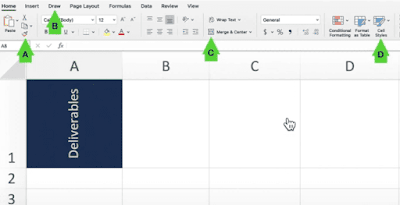• B
• C
• A
• D

1. Which formula correctly counts the number of numeric values in both B4:E4 and G4:I4?

• =COUNT(B4:E4&G4:I4)
• =COUNT(B4:E4,G4:I4)
• =COUNT(B4:E4 G4:I4)
• =COUNT(B4:I4)

1. After activating a chart, which sequence adds a trendline to the chart?

• In the Format group, select Trendline from the Insert Shapes list.
• Click outside the plot area and select Add Trendline
• Click inside the plot and select Forecast.
• Right-click a data series and select Add Trendline.

1. Which Excel add-in will help you find a target result by varying multiple inputs to a formula?

• Goal Seek
• Power Pivot
• Data Analysis
• Solver

1. What tool would you use to prevent the input in a cell of a date outside a specific range?

• Protect Workbook
• Watch Window
• Data Validation
• Filter

1. When you sort a list of numerical value into ascending or descending order, the value in the middle of the list is the _.

• mode
• modulus
• average
• median

1. Which format setting does not change the background appearance of a cell?

• Cell style
• Fill color
• Pattern style
• Font color

1. In Excel, what do most formulas begin with?

• :
• =
• (
• -

1. You need to determine the commission earned by each Sales Rep, based on the Sales amounts in B3:B50 and the Commission rate specified in cell A1. You want to enter a formula in C3 and copy it down to C50. Which formula should you use?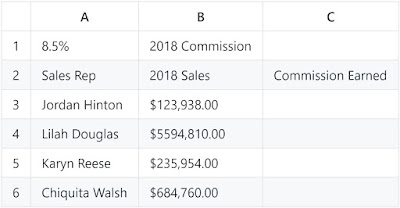• =\$A1*B3
• =\$A\$1*B3
• =A1*\$B3
• =A1*B3

1. If you start a date series by dragging down the fill handle of a single cell that contains the date 12/1/19, what will you get?

• a series of consecutive days following the initial date
• a series of days exactly one month apart
• a series of days identical to the initial date
• a series of days exactly one year apart

1. To discover how many cells in a range contain values that meet a single criterion, use the ___ function.

• COUNT
• SUMIFS
• COUNTA
• COUNTIF

1. Your worksheet has the value 27 in cell B3. What value is returned by the function =MOD (B3,6)?

• 4
• 1
• 5
• 3

1. For an IF function to check whether cell B3 contains a value between 15 and 20 inclusively, what condition should you use?

• OR(B3=>15,B3<=20)
• AND (B3>=15,B3<=20)
• OR(B3>15,B3<20)
• AND(B3>15, B3<20)

1. Which format setting does not change the background appearance of a cell?

• Fill color
• Font Color
• Pattern Style
• Cell Style

1. The charts below are based on the data in cells A3:G5. The chart on the right was created by copying the one on the left. Which ribbon button was clicked to change the layout of the chart on the right?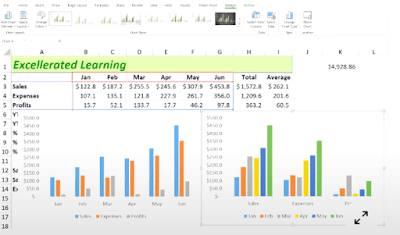• Move Chart
• Switch Row/Column
• Quick Layout
• Change Chart Type

NOTE: We can get the chart on the right side in 2 ways: Switch Row/Column and Change Chart Type
[ Still I feel, Switch Row/Column is more suitable option ]

1. Cell A20 displays an orange background when its value is 5. Changing the value to 6 changes the background color to green. What type of formatting is applied to cell A20?

• Value Formatting
• Cell Style Formatting
• Conditional Formatting
• Tabular format

1. What does this formula do?
`=Sum(Sheet1:Sheet4!D18)`

• It adds data from cell D18 of Sheet1 and cell D18 of Sheet4
• It adds data from cell A1 of Sheet1 and cell D18 of sheet4
• It adds all data in the range A1:D18 in Sheet1, Sheet2, Sheet3 and Sheet4
• It adds data from all D18 cells in Sheet1, Sheet2, Sheet3 and Sheet4

1. What is the term for an expression that is entered into a worksheet cell and begins with an equal sign?

• function
• argument
• formula
• contents

1. How does the appearance of an array formula differ from that of a standard formula?

• In a worksheet cell, array formulas have a small blue triangle in the cell’s upper-right corner.
• A heavy border appears around the range that is occupied by the array formula.
• In the formula bar, an array formula appears surrounded by curly brackets.
• When a cell that contains an array formula is selected, range finders appear on the worksheet around the formula’s precedent cells.

1. In a worksheet, column A contains employee last names, column B contains their middle initials (if any), and column C contains their first names. Which tool can combine the last names, initials, and first names in column D without using a worksheet formula?

• Concatenation
• Columns to Text
• Flash Fill
• AutoFill

1. Which formula returns the value in cell A10 of the worksheet named Budget Variances?

• =‘Budget Variances’!A10
• =‘Budget Variances!A10’
• =“BudgetVariances!A10”
• =“BudgetVariances”!A10

1. Which function returns the leftmost five characters in cell A1?

• =FIND(A1,1,5)
• =SEARCH(A1,5)
• =LEFT(A1,5)
• =A1-RIGHT(A1,LEN(A1)-5)

1. Which function returns TRUE if cell A1 contains a text value?

• =ISALPHA(A1)
• =ISCHAR(A1)
• =ISSTRING(A1)
• =ISTEXT(A1)

1. Which of these functions returns the largest value among all values within the range H2:H30?

• =UPPER(H2:30,1)
• =MAXIMUM(H2:H30)
• =MAX(H2:H30)
• =LARGE(H2:H30,29)

1. You select cell A1, hover the pointer over the cell border to reveal the move icon, then drag the cell to a new location. Which ribbon commands achieve the same result?

• Cut and Fill
• Cut and Paste
• Copy and Transpose
• Copy and Paste

1. You want to add a column to the PivotTable below that shows a 5% bonus for each sales rep. That data does not exists in the original data table. How can you do this without adding more data to the table?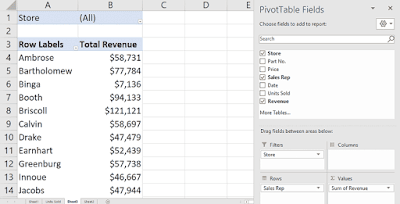• Add a new PivotTable field.
• Add a new Summarize Value By field.

1. You need to determine the commission earned by each Sales rep, based on the Sales amount in B3:B50 and the Commission rate specified in cell A1. You want to enter a formula in C3 and copy it down to C50. Which formula should you use?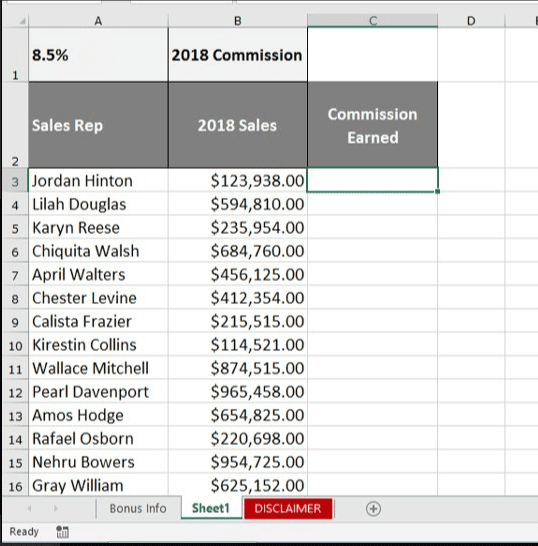• =A1*\$B3
• =A1*B3
• =\$A\$1*B3
• =\$A1*B3

1. The NOW() function returns the current date and time as 43740.665218. Which part of this value indicates the time?

• 6652
• 43740.665218
• 43740
• 665218

1. In the worksheet below, a formula in cell M2 counts the number of projects that start with the letter P. What is this formula?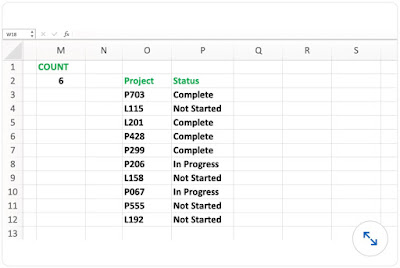• =COUNTIFS(O3:O12,“P”&"*")
• =COUNTIFS(O3:O12,“P”&?)
• =COUNTIFS(O3:O12,“P?”)
• =COUNTIFS(O3:O12,P*)

CREDITS: (Source)

&